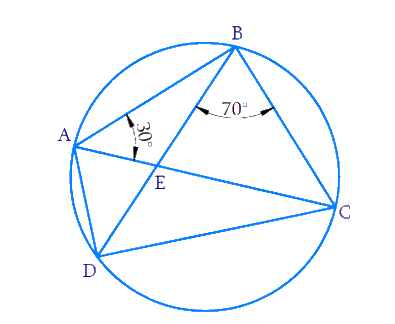# Ex.10.5 Q6 Circles Solution - NCERT Maths Class 9

Go back to  'Ex.10.5'

## Question

\begin {align} {ABCD}\end {align} is a cyclic quadrilateral whose diagonals intersect at a point \begin {align} {E. }\end {align}If \begin {align} \angle {DBC}=70^{\circ}, \quad \angle {BAC}=30^{\circ} \end {align} find \begin {align} \angle {BCD}. \end {align} Further if \begin {align} {AB=BC,} \end {align} find \begin {align} \angle {ECD.} \end {align}

Video Solution
Circles
Ex 10.5 | Question 6

## Text Solution

What is known?

\begin {align} {ABCD}\end {align} is cyclic quadrilateral. \begin {align} {DBC}=70^\circ \end {align} and \begin {align} {BAC}=30^\circ. \end {align} \begin {align}{AB = BC.}\end {align}

What is unknown?

Value of \begin {align} \angle {BCD} \text { and } \angle {ECD} \end {align}

Reasoning:

• A quadrilateral \begin {align} {ABCD}\end {align} is called cyclic if all the four vertices of it lie on a circle.
• The sum of either pair of opposite angles of a cyclic quadrilateral is $$180^\circ.$$
• Sum of angles in a triangle is $$180^\circ.$$
• Angles in the same segment are equal.

Steps:

Based on the data given, draw the figure.In the triangles $${ABD}$$ and $${BCD,}$$ \begin {align} \angle {CAD}=\angle {CBD}=70^{\circ}. \end {align} (Angles in the same segment)

So \begin {align} \angle {BAD}=30^{\circ}+70^{\circ}=100^{\circ} \end {align}

Since \begin {align} {ABCD }\end {align}is a cyclic quadrilateral, the sum of either pair of opposite angles of cyclic quadrilateral is $$180^{\circ}.$$

\begin{align} \angle {BAD}+ \angle {BCD}&=180^{\circ} \\ \angle {BCD} &=180^{\circ}-100^{\circ} \\&=80^{\circ} \end{align}

Also given \begin{align} {AB = BC.}\end{align}

So, \begin {align} \angle {BCA}=\angle {BAC}=30^{\circ} \end {align} (Base angles of isosceles triangle are equal)

\begin{align} ∴ \quad \angle {ECD} &=\angle {BCD}-\angle {BCA} \\ &=80^{\circ}-30^{\circ} \\ &=50^{\circ} \end{align}

Video Solution
Circles
Ex 10.5 | Question 6

Learn from the best math teachers and top your exams

• Live one on one classroom and doubt clearing
• Practice worksheets in and after class for conceptual clarity
• Personalized curriculum to keep up with school